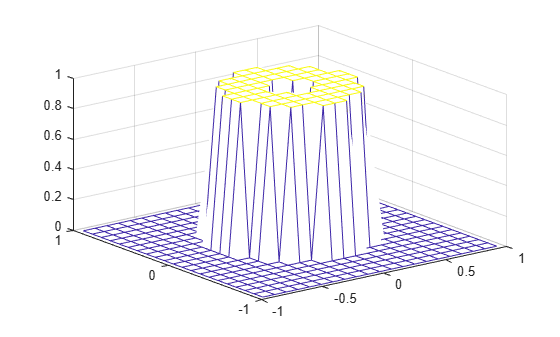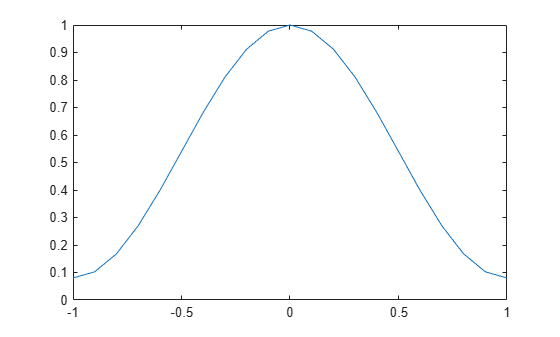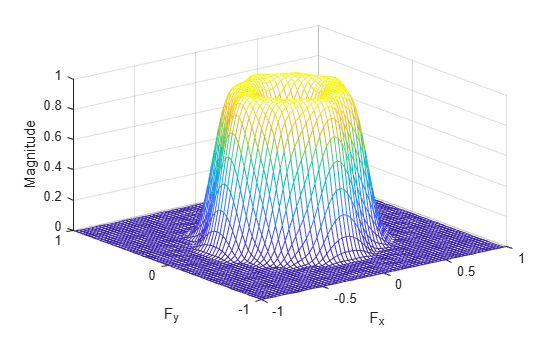fwind1

2-D FIR filter using 1-D window method

Description

The fwind1 function designs 2-D FIR filters using the window method. fwind1 uses a 1-D window specification to design a 2-D FIR filter based on the desired frequency response. fwind1 works with 1-D windows only. Use fwind2 to work with 2-D windows.

You can apply the 2-D FIR filter to images by using the filter2 function.

example

h = fwind1(Hd,win) creates a 2-D FIR filter h based on the desired frequency response Hd. The fwind1 function uses the 1-D window win to form an approximately circularly symmetric 2-D window using Huang's method.

h = fwind1(Hd,win1,win2) uses two 1-D windows, win1 and win2, to create a separable 2-D window.

h = fwind1(f1,f2,Hd,___) enables you to specify the desired frequency response Hd at arbitrary frequencies f1 and f2 along the x- and y-axes.

Examples

collapse all

This example shows how to design an approximately circularly symmetric two-dimensional bandpass filter using a 1-D window method.

Create the frequency range vectors f1 and f2 using freqspace. These vectors have length 21.

[f1,f2] = freqspace(21,'meshgrid');

Compute the distance of each position from the center frequency.

r = sqrt(f1.^2 + f2.^2);

Create a matrix Hd that contains the desired bandpass response. In this example, the desired passband is between 0.1 and 0.5 (normalized frequency, where 1.0 corresponds to half the sampling frequency, or $\pi$ radians).

Hd = ones(21);
Hd((r<0.1)|(r>0.5)) = 0;

Display the ideal bandpass response.

colormap(parula(64))
mesh(f1,f2,Hd)Design the 1-D window. This example uses a Hamming window of length 21.

win = 0.54 - 0.46*cos(2*pi*(0:20)/20);

Plot the 1-D window.

figure
plot(linspace(-1,1,21),win);Using the 1-D window, design the filter that best produces this frequency response

h = fwind1(Hd,win);

Display the actual frequency response of this filter.

freqz2(h)Input Arguments

collapse all

Desired frequency response, specified as a numeric matrix. Hd is sampled at equally spaced points between -1.0 and 1.0 (in normalized frequency, where 1.0 corresponds to half the sampling frequency, or π radians) along the x and y frequency axes. For accurate results, create Hd by using the freqspace function.

Data Types: single | double | int8 | int16 | int32 | int64 | uint8 | uint16 | uint32 | uint64

1-D window, specified as a numeric matrix. If you have Signal Processing Toolbox™ software, then you can specify win using windows such as hamming (Signal Processing Toolbox), hann (Signal Processing Toolbox), bartlett (Signal Processing Toolbox), blackman (Signal Processing Toolbox), kaiser (Signal Processing Toolbox), or chebwin (Signal Processing Toolbox).

Data Types: single | double

1-D window, specified as a numeric matrix.

Data Types: single | double

1-D window, specified as a numeric matrix.

Data Types: single | double

Desired frequency along the x-axis. The frequency vector should be in the range [-1, 1], where 1.0 corresponds to half the sampling frequency, or π radians.

Data Types: single | double

Desired frequency along the y-axis. The frequency vector should be in the range [-1, 1], where 1.0 corresponds to half the sampling frequency, or π radians.

Data Types: single | double

Output Arguments

collapse all

2-D FIR filter, returned as a numeric matrix. The length of the window controls the size of the resulting filter.

• If you specify a single window win of length n, then the size of h is n-by-n.

• If you specify two windows win1 and win2 of length n and m respectively, then the size of h is m-by-n.

If Hd is of data type single, then h is of data type single. Otherwise, h is of data type double.

Data Types: single | double

Algorithms

The fwind1 function takes a one-dimensional window specification and forms an approximately circularly symmetric two-dimensional window using Huang's method,

$w\left({n}_{1},{n}_{2}\right)={w\left(t\right)|}_{t=\sqrt{{n}_{{}_{1}}^{2}+{n}_{2}^{2}}},$

where w(t) is the one-dimensional window and w(n1,n2) is the resulting two-dimensional window.

Given two windows, the fwind1 function forms a separable two-dimensional window:

$w\left({n}_{1},{n}_{2}\right)={w}_{1}\left({n}_{1}\right){w}_{2}\left({n}_{2}\right).$

The fwind1 function calls the fwind2 with the desired frequency response Hd and the two-dimensional window. The fwind2 function calculates h using an inverse Fourier transform and multiplication by the two-dimensional window:

${h}_{d}\left({n}_{1},{n}_{2}\right)=\frac{1}{{\left(2\pi \right)}^{2}}{\int }_{-\pi }^{\pi }{\int }_{-\pi }^{\pi }{H}_{d}\left({\omega }_{1},{\omega }_{2}\right){e}^{j{\omega }_{1}{n}_{1}}{e}^{j{\omega }_{2}{n}_{2}}d{\omega }_{1}d{\omega }_{2}$

$h\left({n}_{1},{n}_{2}\right)={h}_{d}\left({n}_{1},{n}_{2}\right)w\left({n}_{1},{n}_{2}\right)$

 Lim, Jae S., Two-Dimensional Signal and Image Processing, Englewood Cliffs, NJ, Prentice Hall, 1990.